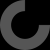MakerBot Print is our newest print-prepration software, which supports native CAD files and STL assemblies,
allows you to interact with all your printers via the Cloud, and many other exciting new features.

# Report as inappropriate

HI
first you'll need to install PiHole and the status script also uses the Luma core package that will also need to be installed. I find this the easiest to use and also it handles the screen rotation.

``````    #!/usr/bin/env python
# -*- coding: utf-8 -*-
# PYTHON_ARGCOMPLETE_OK

"""
Clock Ported from: https://gist.github.com/TheRayTracer/dd12c498e3ecb9b8b47f#file-clock-py
"""

import math
import time
import datetime
import subprocess
import json
import requests
import RPi.GPIO as GPIO

GPIO.setmode(GPIO.BCM)
GPIO.setwarnings(False)
GPIO.setup(23,GPIO.OUT)
GPIO.output(23,GPIO.HIGH)

from luma.core.render import canvas
from luma.core.interface.serial import i2c
from luma.oled.device import ssd1306

device = ssd1306(serial,width=128, height=32, rotate=3)

def posn(angle, arm_length):
return (dx, dy)

def main():
today_last_time = "Unknown"
while True:

now = datetime.datetime.now()
today_date = now.strftime("%d/%m")
today_time = now.strftime("%H:%M")
GPIO.output(23,GPIO.LOW)

if today_time != today_last_time:
# Pi Hole data!
try:
r = requests.get(api_url)
DNSQUERIES = data['dns_queries_today']
CLIENTS = data['unique_clients']
except:
time.sleep(.2)
continue

GPIO.output(23,GPIO.HIGH)
today_last_time = today_time
with canvas(device) as draw:
now = datetime.datetime.now()
today_date = now.strftime("%d/%m")

margin = 0

cx = 16
cy = 15
ecy = 30

left = cx - cy
right = cx + cy

hrs_angle = 270 + (30 * (now.hour + (now.minute / 60.0)))
hrs = posn(hrs_angle, cy - margin - 7)

min_angle = 270 + (6 * now.minute)
mins = posn(min_angle, cy - margin - 2)

sec_angle = 270 + (6 * now.second)
secs = posn(sec_angle, cy - margin - 2)

draw.ellipse((left + margin, margin, right - margin, ecy), outline="white")

draw.line((cx, cy, cx + hrs, cy + hrs), fill="white")
draw.line((cx, cy, cx + mins, cy + mins), fill="white")
#draw.line((cx, cy, cx + secs, cy + secs), fill="red")
draw.ellipse((cx - 2, cy - 2, cx + 2, cy + 2), fill="white", outline="white")

draw.text((( margin), ecy + 8), today_time, fill="yellow")
draw.text((( margin), ecy +16), today_date, fill="yellow")

bstart = 30

cstart= 52
draw.text((margin,ecy + cstart),"Users"                 , fill="white")
draw.text((margin,ecy + cstart+8),str(CLIENTS)  , fill="white")

dstart = 74
draw.text((margin,ecy + dstart),chr(35)+"DNS"           , fill="white")
draw.text((margin,ecy + dstart+8), str(DNSQUERIES)      , fill="white")

time.sleep(0.1)
GPIO.output(23,GPIO.HIGH)

if __name__ == "__main__":
try:
main()
except KeyboardInterrupt:
pass``````